# 如何通俗易懂地解释卷积？

卷积这个概念，很早以前就学过，但是一直没有搞懂。教科书上通常会给出定义，给出很多性质，也会用实例和图形进行解释，但究竟为什么要这么设计，这么计算，背后的意义是什么，往往语焉不详。一个公式倘若倘若给不出结合实际的直观的通俗的解释（也就是背后的“物理”意义），就觉得少了点什么，觉得不是真的懂了。

教科书上一般定义函数  $f, g$  的卷积  $f * g(n)$  如下:

连续形式:

$(f * g)(n)=\int_{-\infty}^{\infty} f(\tau) g(n-\tau) d \tau$

离散形式:

$(f * g)(n)=\sum_{\tau=-\infty}^{\infty} f(\tau) g(n-\tau)$

这两个式子有一个共同的特征：

$n=\tau+(n-\tau)$

这个特征有什么意义？

我们令  $x=\tau, y=n-\tau$  ，那么  $x+y=n$  就是下面这些直线: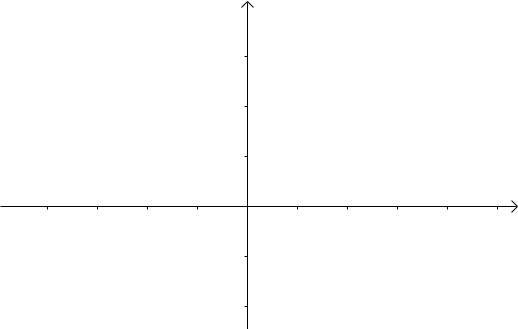如果遍历这些直线，就好比，把毛巾沿着角卷起来：我有两枚骰子，把这两枚骰子都抛出去，求两枚骰子点数加起来为 4 的概率是多少?

这里问题的关键是，两个骰子加起来要等于 4，这正是卷积的应用场景。

我们把骰子各个点数出现的概率表示出来: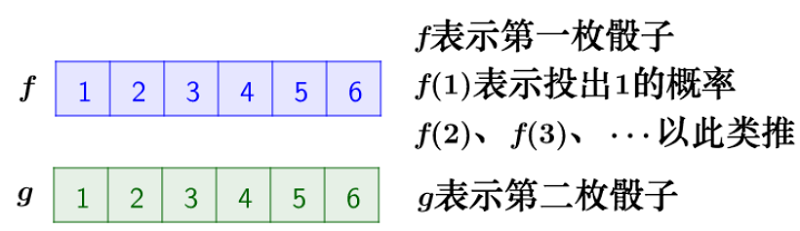那么，两枚骰子点数加起来为4的情况有：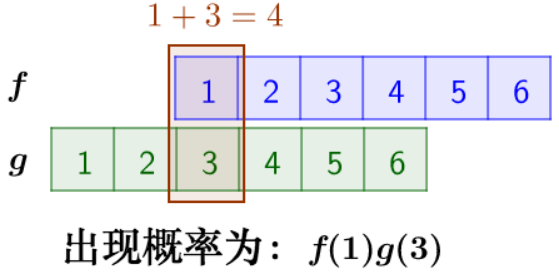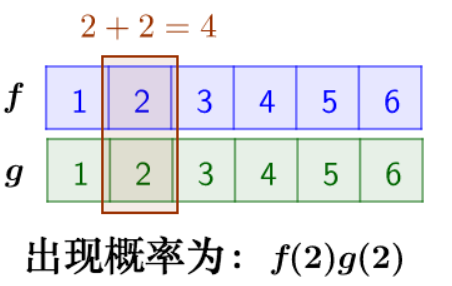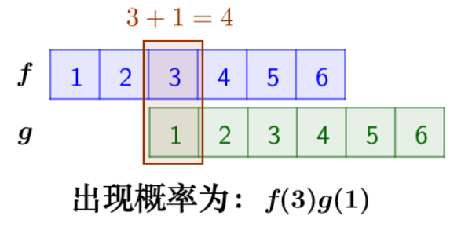因此，两枚骰子点数加起来为4的概率为：

$f(1) g(3)+f(2) g(2)+f(3) g(1)$

符合卷积的定义，把它写成标准的形式就是：

$(f * g)(4)=\sum \limits_{m=1}^{3} f(4-m) g(m)$

楼下早点铺子生意太好了，供不应求，就买了一台机器，不断的生产馒头。

假设馒头的生产速度是  $f(t)$ ，那么一天后生产出来的馒头总量为:

$\int_{0}^{24} f(t) d t$

馒头生产出来之后，就会慢慢腐败，假设腐败函数为 g(t) ，比如，10个漫头，24小时会腐败：

$10 * g(t)$

想想就知道，第一个小时生产出来的馒头，一天后会经历24小时的腐败，第二个小时生产出来的馒 头，一天后会经历 23 小时的腐败。
如此，我们可以知道，一天后，馒头总共腐败了:

$\int_{0}^{24} f(t) g(24-t) d t$

这就是连续的卷积。

posted @ 2022-01-13 15:30  Learner-  阅读(183)  评论(0编辑  收藏  举报